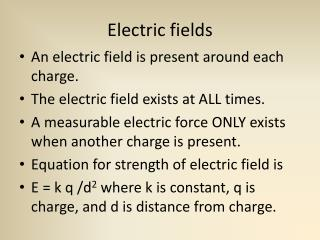DownloadDownload PresentationElectric fields

# Electric fields

Download Presentation## Electric fields

- - - - - - - - - - - - - - - - - - - - - - - - - - - E N D - - - - - - - - - - - - - - - - - - - - - - - - - - -
##### Presentation Transcript

1. Electric fields • An electric field is present around each charge. • The electric field exists at ALL times. • A measurable electric force ONLY exists when another charge is present. • Equation for strength of electric field is • E = k q /d2 where k is constant, q is charge, and d is distance from charge.

2. Electric fields

3. Electric fields

5. Electric fields • What is the strength of an electric field created by a single proton at a distance of 0.25 meters? • What is the strength of the electric field created by 10,000 electrons at a distance of 0.0005 m?

6. Electric fields practice • What is the strength of an electric field created by a 30 protons at a distance of 0.003 meters? • At what distance from a charge of 6 µC will an electron experience a field strength of 2.5 N/C ?

7. Practice • Calculate the charge present if a field strength of 3500 N/C is experienced at a distance of 0.38 m from a point charge. • How many electrons are present on a charged object if a field strength of 1200 N/C is measured at a distance of 0.15 meters?

8. Charges in electric fields • If an electron is placed in an electric field where the field strength is 600 N/C, what force will be experienced by the electron? • How much force would be experienced by a 7 C charge at a point where the field strength is 2000 N/C?

9. Electric potential energy q E d m g h Gravitational PE =mgh Electric PE = qEd Zero potential

10. Electric potential energy • How much potential energy does an electron have when it is moved 0.1 meters against an electric field with a strength of 200 N/C? • How much charge will an object have if it has a potential energy of 1.5 Joules when moved 0.05 meters against a field with strength of 5 N/C?

11. Electric potential • Electric potential does not have a counterpart in gravitational fields. • Electric potential is the electric potential energy of a single charge. • It is calculated by dividing the electric potential energy by the charge. • Given the name voltage V = qEd/q = Ed

12. Electric potential • How many volts are required to move an electron 0.01 meters against an 2 N/C electric field? • How far will a 1.5 volt battery move a charge against a 2 N/C electric field?

13. Electric potential and work • Recall that work and potential energy are equivalent. • If PE = qEd = W, and V = Ed, then by substituting V for Ed, we get W = qV. • How much work is required to push an electron through a 1.5 volt battery? • How is this work accomplished?

14. Capacitors • A capacitor stores energy in an electric field. V = Ed

15. Capacitors • A capacitor is an energy storage device. Basically a rolled up parallel plate capacitor.

16. Charges in electric fields • If an electron is placed in an electric field where the field strength is 4000 N/C, what force will be experienced by the electron? • How much force would be experienced by a 2.5 C charge at a point where the field strength is 5000 N/C?

17. Practice • Calculate the electric field strength of a parallel plate capacitor separated by 0.006 meters and connected to a 12 volt battery. • How many volts are required to give parallel plates separated by 0.0025 meters an electric field strength of 2.7 N/C?